##All rectangular hyperbolas tangent to four lines

Problem: Construct all rectangular hyperbolas tangent to four given lines determined by the sides of a quadrilateral q = A1A2A3A4 (may be convex or not).
The problem admits either two solutions or no solution at all. Since a triangle whose sides are tangent to a rectangular hyperbola must be obtuse-angled, the problem has solutions only if all partial triangles formed out of the four given points are obtuse-angled.
By partial triangles I mean the four triangles formed by selecting three sides of q. The picture below shows such a case where two hyperbolas exist. In addition it shows the tangent to the four sides parabola, which is always uniquely determined by q.
When the existence condition, described above, is valid, the crucial fact is that the conjugate circles of the four partial triangles are all members of a circle bundle of intersecting type. The two common points O1, O2 of this circle bundle belong to the Newton-line of q, which is parallel to the axis of the tangent parabola of q.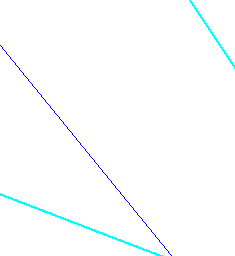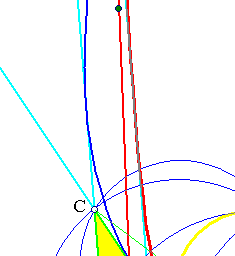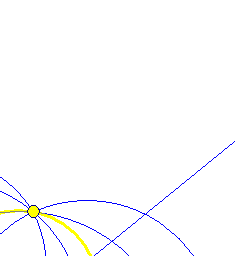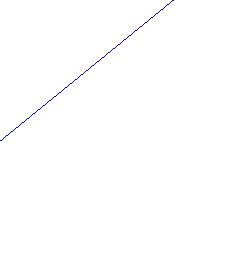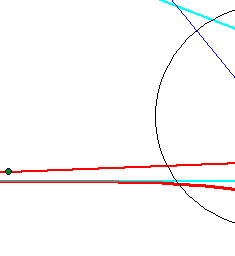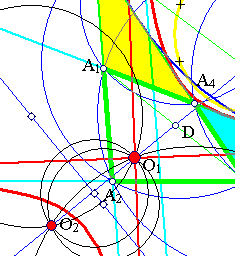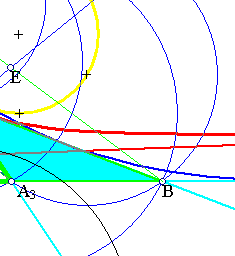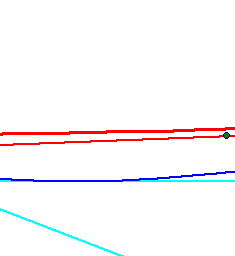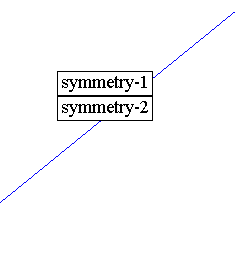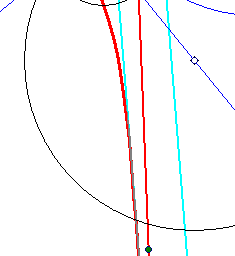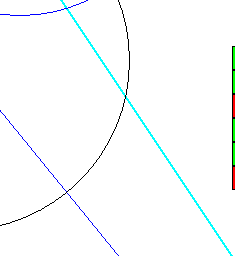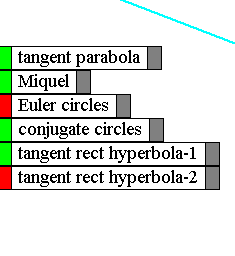A case where the construction of tangent hyperbolas is impossible is shown in RectHypeImpossible.html .

Euler.html
EulerCircleProperty.html
FourEulerCircles.html
HyperbolaRectangular.html
Miquel_Point.html
NinePointsConic.html
OrthoRectangular.html
RectHypeCircumscribed.html
RectHypeImpossible.html
RectHyperbolaTriaInscribed.html
RectHypeThroughFourPts.html
Tangent4Lines.html